# RS Aggarwal Solutions for Class 7 Maths Exercise 5A Chapter 5 Exponents

RS Aggarwal Solutions for Class 7 Maths Exercise 5A Chapter 5 Exponents, Students can download the PDF which is given here. Students are going to learn about Exponents, the product of a rational number multiplied several times by itself can be expressed in the same notation. It contains other topics like Laws of Exponents. Expert tutors at BYJU’S have prepared the RS Aggarwal Solutions for Class 7 Maths Chapter 5 Exponents. Students gain more knowledge by referring to RS Aggarwal Class 7 Solutions.

## Download the PDF of RS Aggarwal Solutions For Class 7 Maths Chapter 5 Exponents – Exercise 5A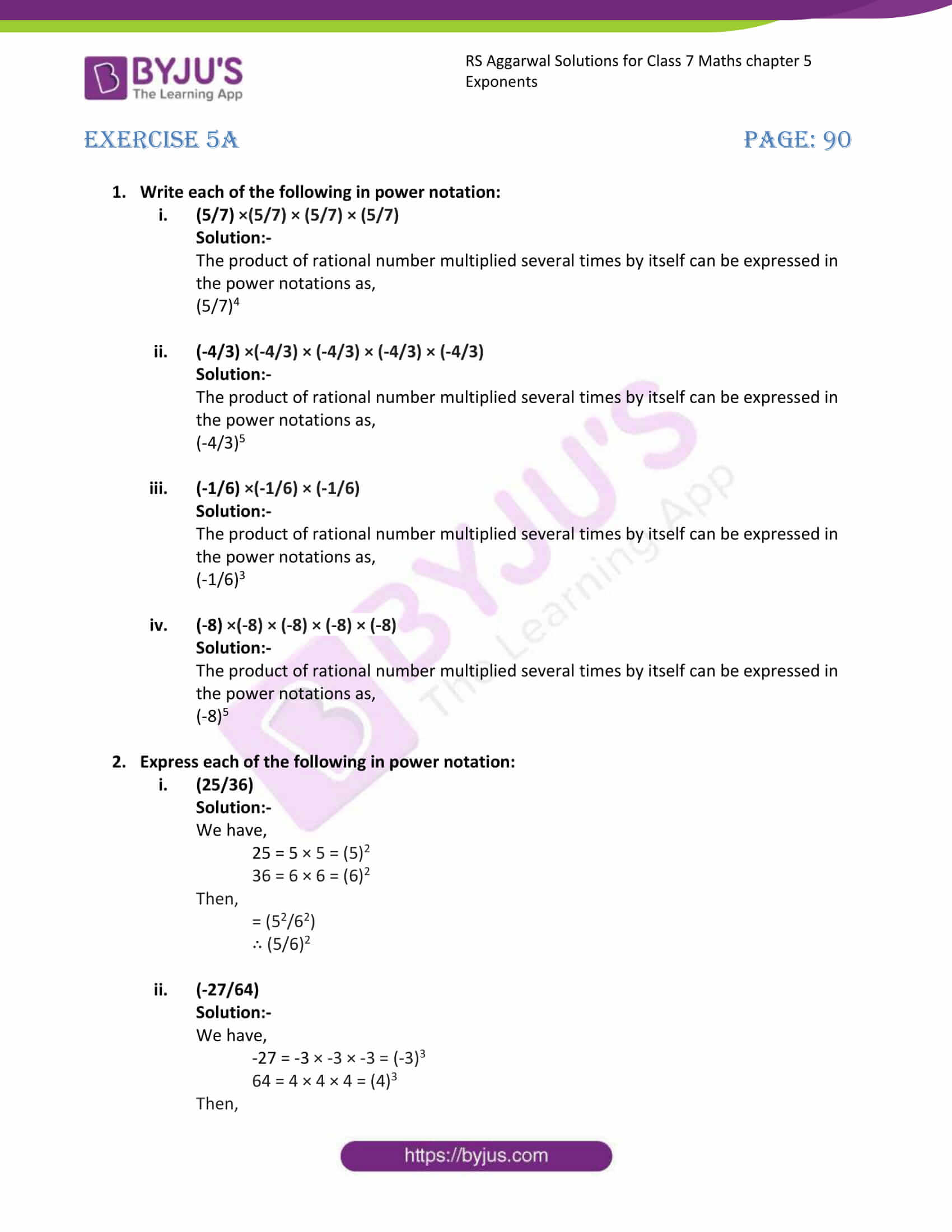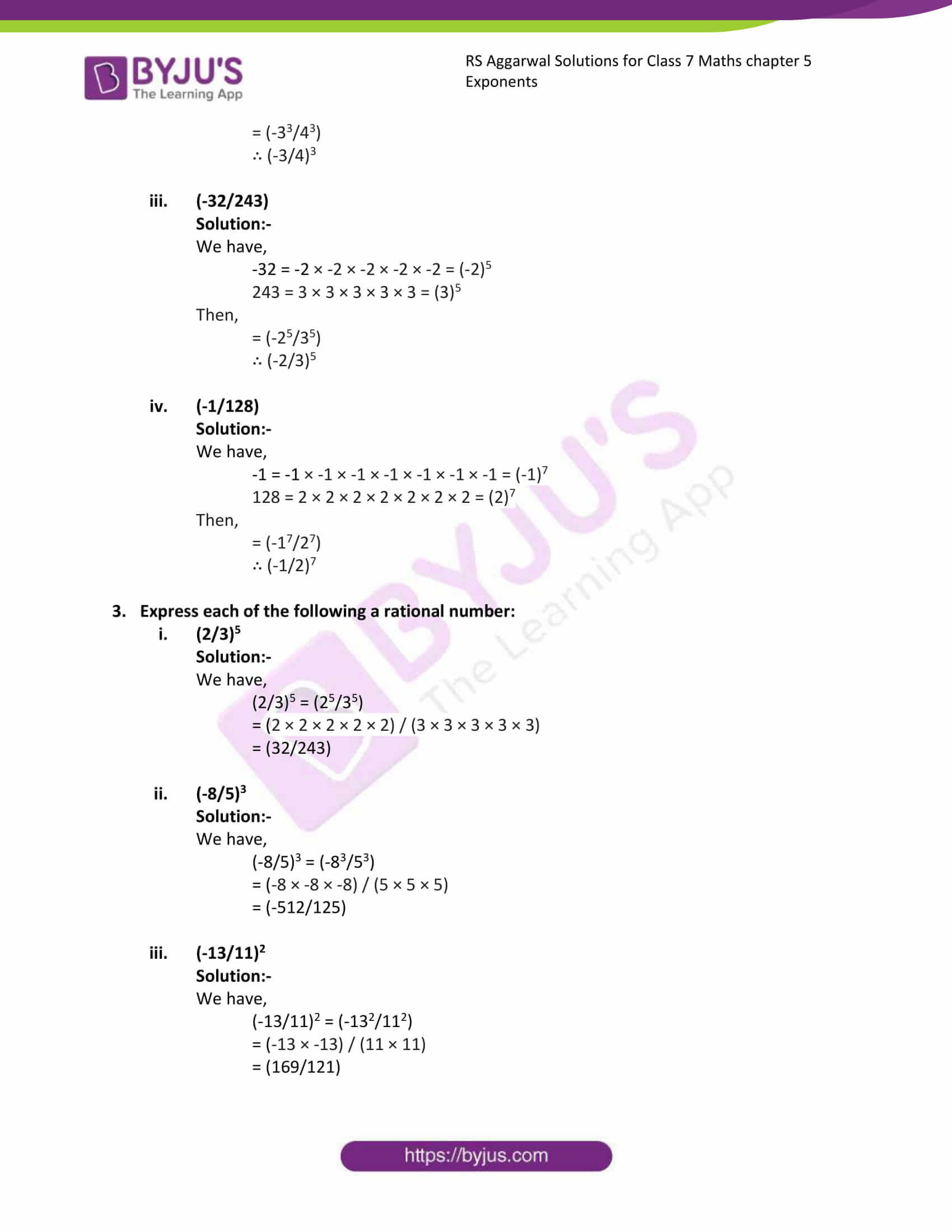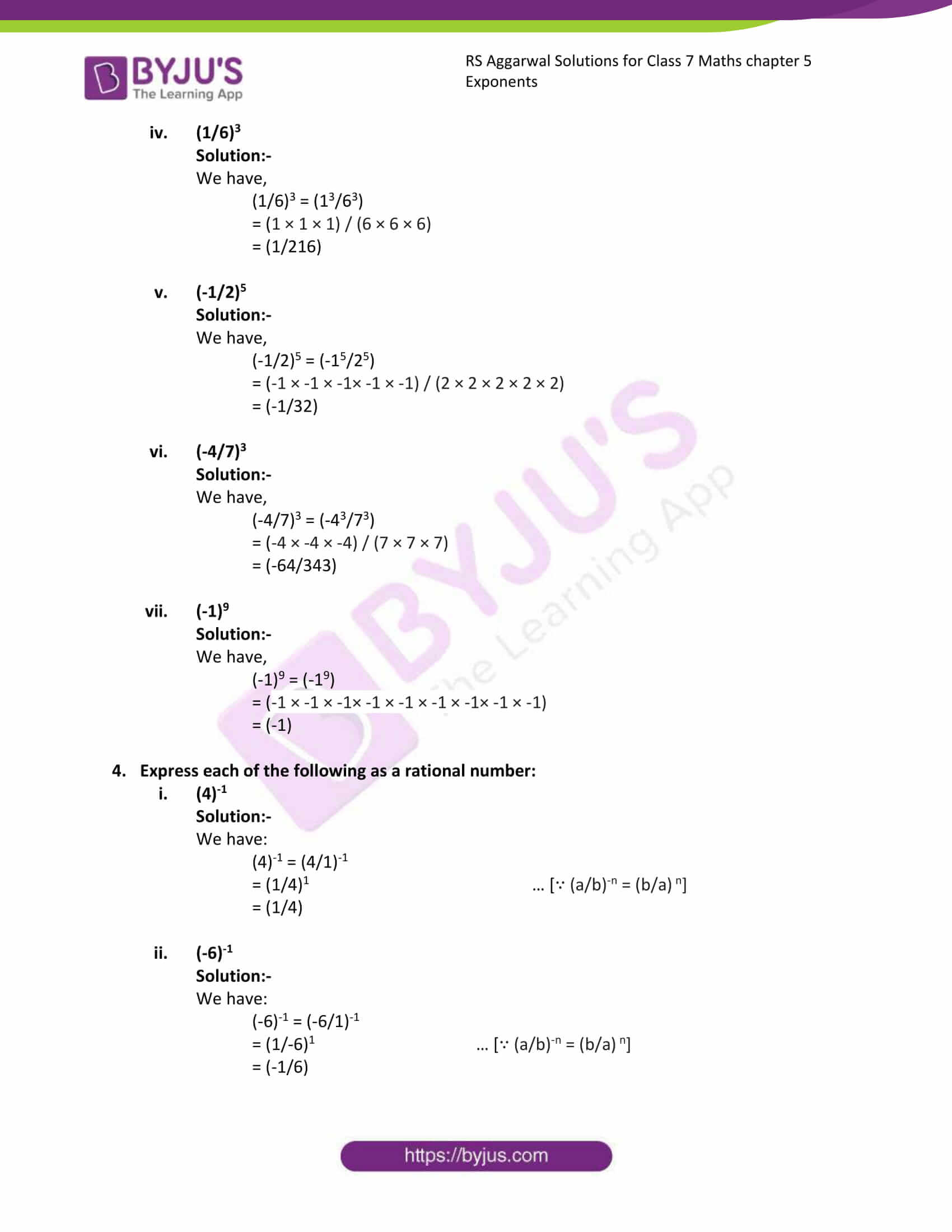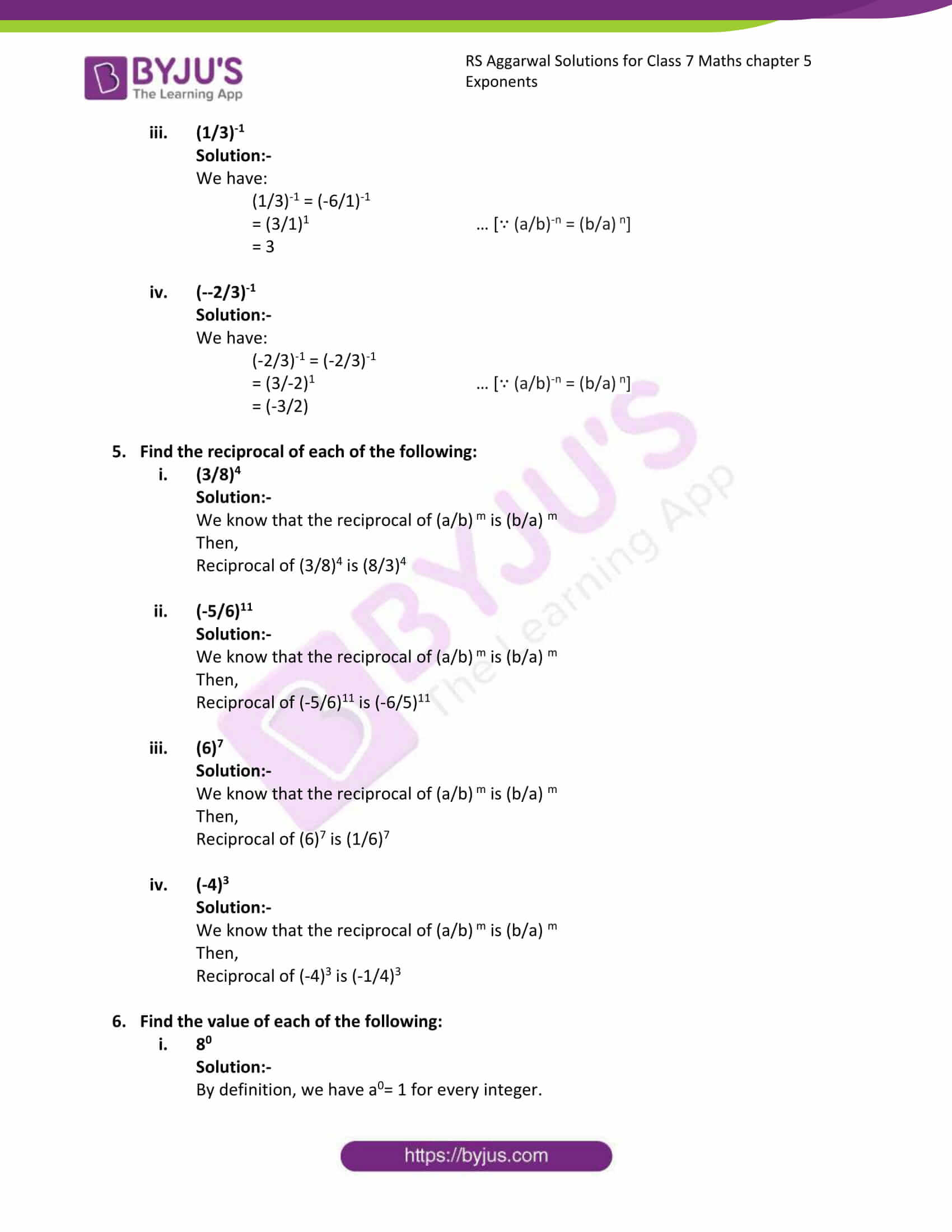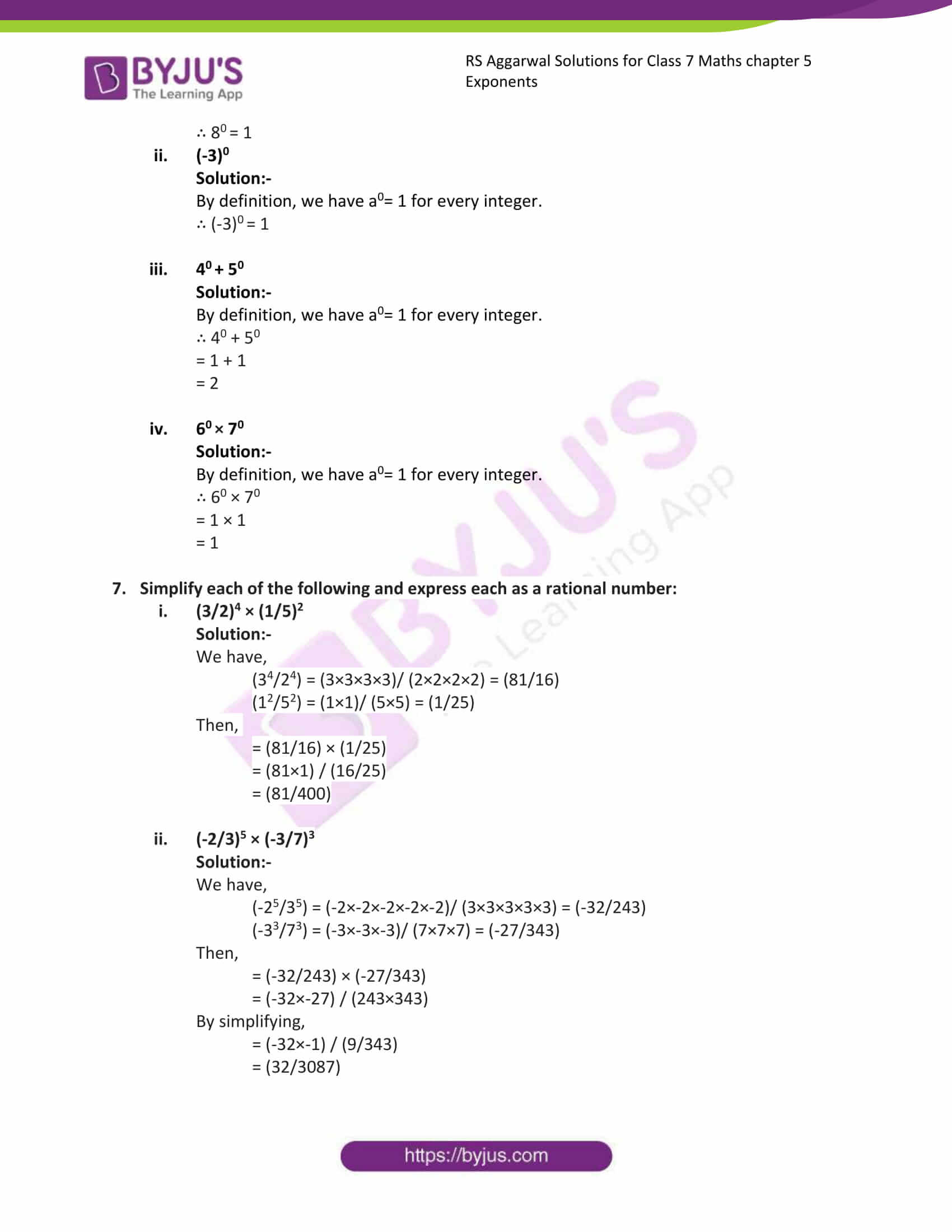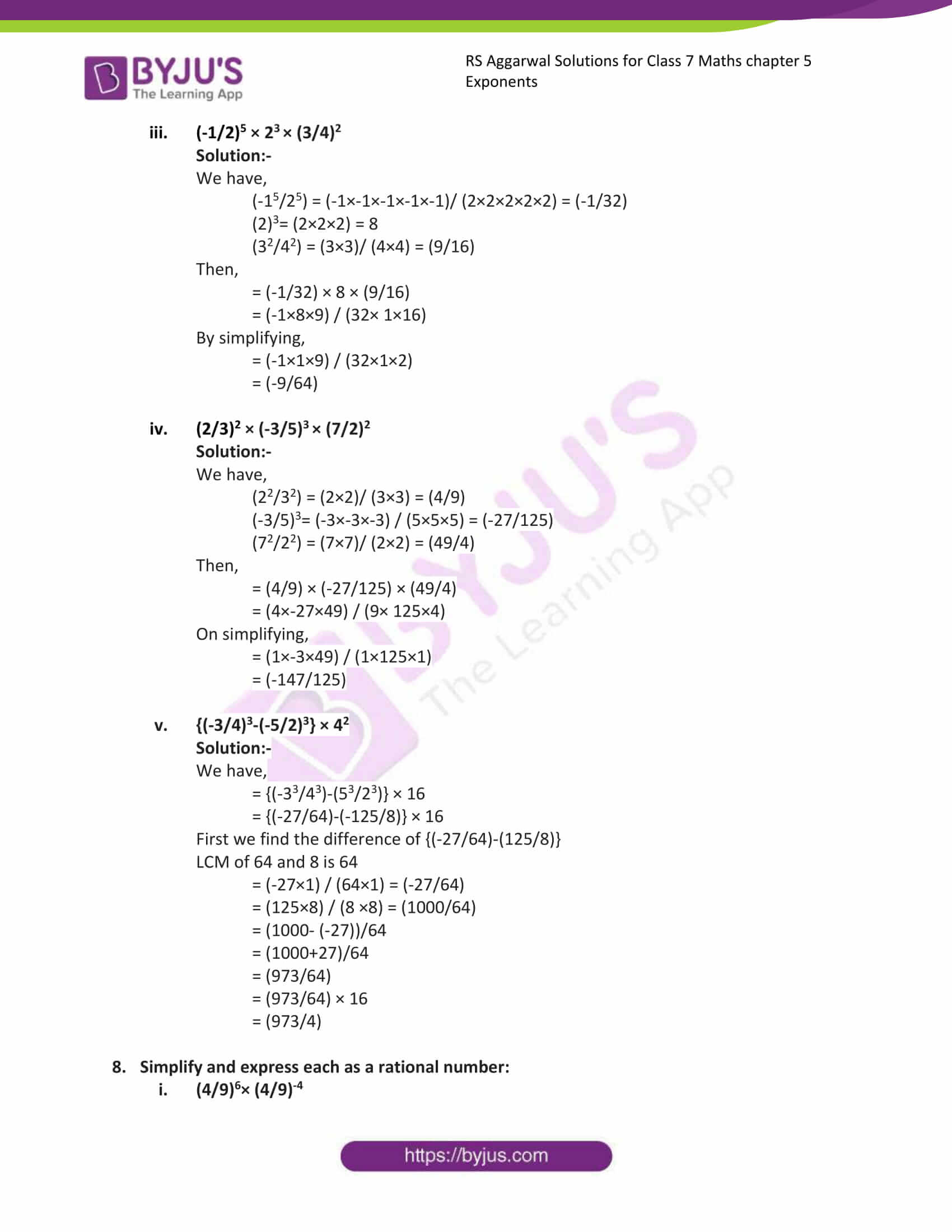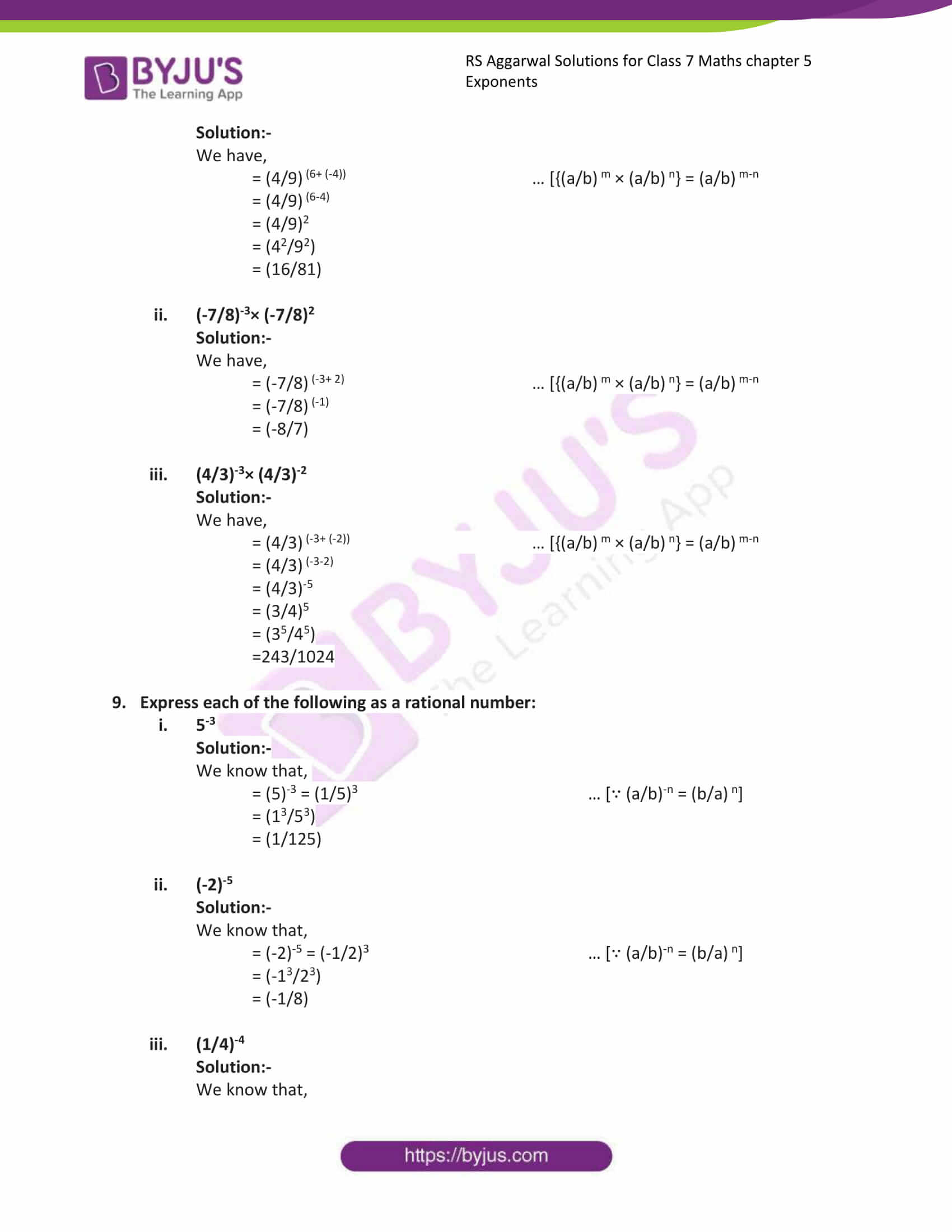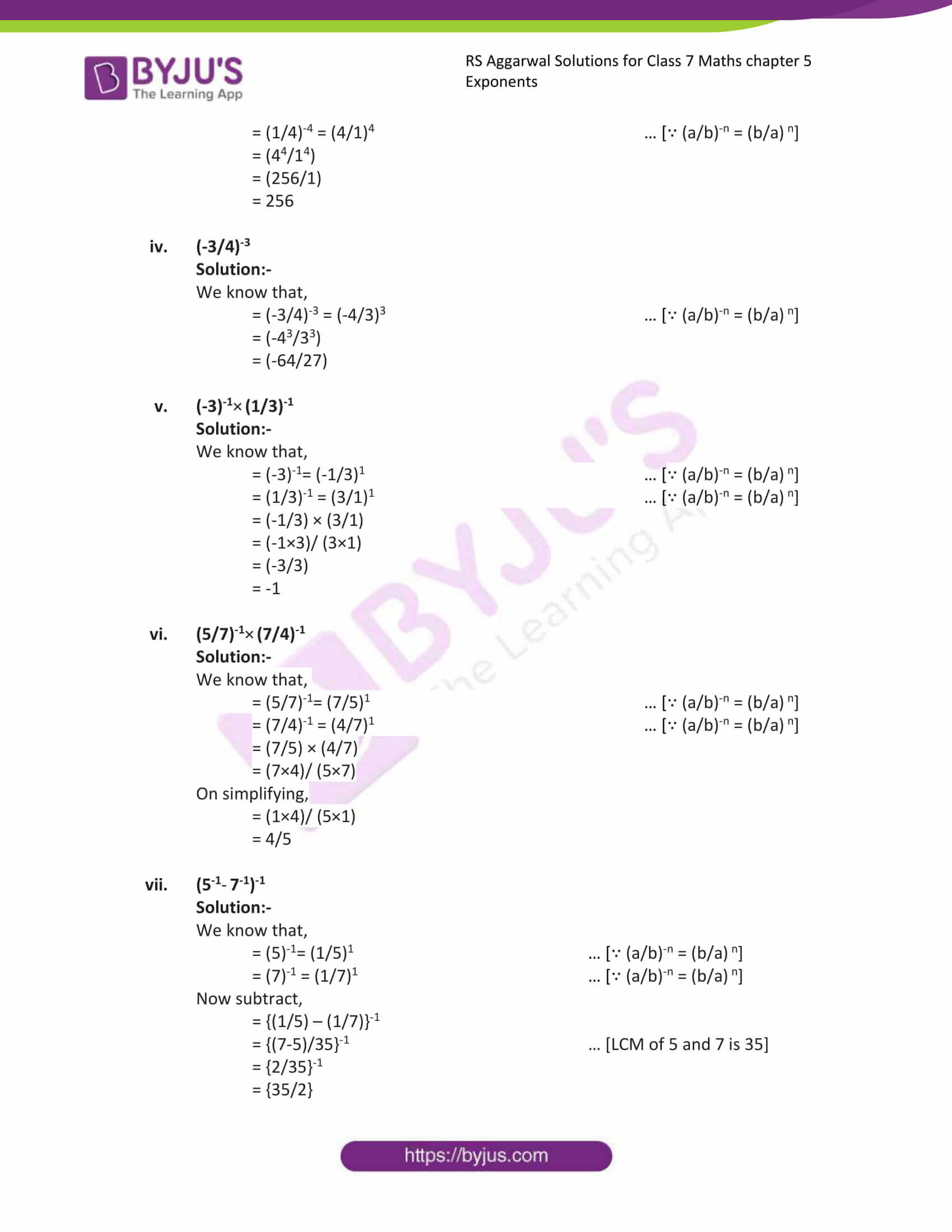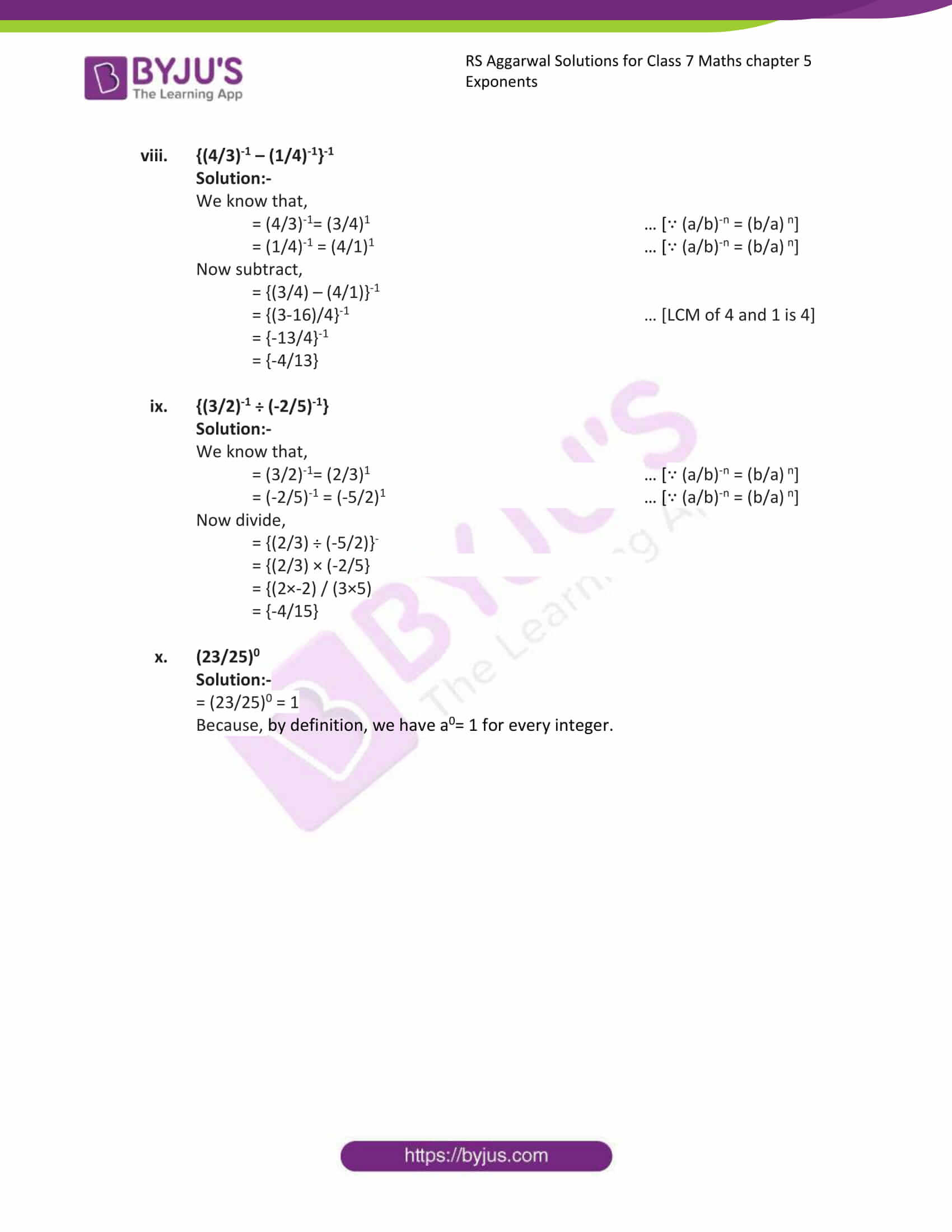### Access answers to Maths RS Aggarwal Solutions for Class 7 Chapter 5 – Exponents Exercise 5A

1. Write each of the following in power notation:

(i) (5/7) ×(5/7) × (5/7) × (5/7)

Solution:-

The product of rational number multiplied several times by itself can be expressed in the power notations as,

(5/7)4

(ii) (-4/3) ×(-4/3) × (-4/3) × (-4/3) × (-4/3)

Solution:-

The product of rational number multiplied several times by itself can be expressed in the power notations as,

(-4/3)5

(iii) (-1/6) ×(-1/6) × (-1/6)

Solution:-

The product of rational number multiplied several times by itself can be expressed in the power notations as,

(-1/6)3

(iv) (-8) ×(-8) × (-8) × (-8) × (-8)

Solution:-

The product of rational number multiplied several times by itself can be expressed in the power notations as,

(-8)5

2. Express each of the following in power notation:

(i) (25/36)

Solution:-

We have,

25 = 5 × 5 = (5)2

36 = 6 × 6 = (6)2

Then,

= (52/62)

∴ (5/6)2

(ii)(-27/64)

Solution:-

We have,

-27 = -3 × -3 × -3 = (-3)3

64 = 4 × 4 × 4 = (4)3

Then,

= (-33/43)

∴ (-3/4)3

(iii) (-32/243)

Solution:-

We have,

-32 = -2 × -2 × -2 × -2 × -2 = (-2)5

243 = 3 × 3 × 3 × 3 × 3 = (3)5

Then,

= (-25/35)

∴ (-2/3)5

(iv) (-1/128)

Solution:-

We have,

-1 = -1 × -1 × -1 × -1 × -1 × -1 × -1 = (-1)7

128 = 2 × 2 × 2 × 2 × 2 × 2 × 2 = (2)7

Then,

= (-17/27)

∴ (-1/2)7

3. Express each of the following a rational number:

(i) (2/3)5

Solution:-

We have,

(2/3)5 = (25/35)

= (2 × 2 × 2 × 2 × 2) / (3 × 3 × 3 × 3 × 3)

= (32/243)

(i) (-8/5)3

Solution:-

We have,

(-8/5)3 = (-83/53)

= (-8 × -8 × -8) / (5 × 5 × 5)

= (-512/125)

(ii) (-13/11)2

Solution:-

We have,

(-13/11)2 = (-132/112)

= (-13 × -13) / (11 × 11)

= (169/121)

(iii) (1/6)3

Solution:-

We have,

(1/6)3 = (13/63)

= (1 × 1 × 1) / (6 × 6 × 6)

= (1/216)

(v) (-1/2)5

Solution:-

We have,

(-1/2)5 = (-15/25)

= (-1 × -1 × -1× -1 × -1) / (2 × 2 × 2 × 2 × 2)

= (-1/32)

(vi) (-4/7)3

Solution:-

We have,

(-4/7)3 = (-43/73)

= (-4 × -4 × -4) / (7 × 7 × 7)

= (-64/343)

(vii) (-1)9

Solution:-

We have,

(-1)9 = (-19)

= (-1 × -1 × -1× -1 × -1 × -1 × -1× -1 × -1)

= (-1)

4. Express each of the following as a rational number:

(i) (4)-1

Solution:-

We have:

(4)-1 = (4/1)-1

= (1/4)1 … [∵ (a/b)-n = (b/a) n]

= (1/4)

(ii) (-6)-1

Solution:-

We have:

(-6)-1 = (-6/1)-1

= (1/-6)1 … [∵ (a/b)-n = (b/a) n]

= (-1/6)

(iii) (1/3)-1

Solution:-

We have:

(1/3)-1 = (-6/1)-1

= (3/1)1 … [∵ (a/b)-n = (b/a) n]

= 3

(iv) (–2/3)-1

Solution:-

We have:

(-2/3)-1 = (-2/3)-1

= (3/-2)1 … [∵ (a/b)-n = (b/a) n]

= (-3/2)

5. Find the reciprocal of each of the following:

(i) (3/8)4

Solution:-

We know that the reciprocal of (a/b) m is (b/a) m

Then,

Reciprocal of (3/8)4 is (8/3)4

(ii) (-5/6)11

Solution:-

We know that the reciprocal of (a/b) m is (b/a) m

Then,

Reciprocal of (-5/6)11 is (-6/5)11

(iii) (6)7

Solution:-

We know that the reciprocal of (a/b) m is (b/a) m

Then,

Reciprocal of (6)7 is (1/6)7

(iv) (-4)3

Solution:-

We know that the reciprocal of (a/b) m is (b/a) m

Then,

Reciprocal of (-4)3 is (-1/4)3

6. Find the value of each of the following:

(i) 80

Solution:-

By definition, we have a0= 1 for every integer.

∴ 80 = 1

(ii) (-3)0

Solution:-

By definition, we have a0= 1 for every integer.

∴ (-3)0 = 1

(iii) 40 + 50

Solution:-

By definition, we have a0= 1 for every integer.

∴ 40 + 50

= 1 + 1

= 2

(iv) 60 × 70

Solution:-

By definition, we have a0= 1 for every integer.

∴ 60 × 70

= 1 × 1

= 1

7. Simplify each of the following and express each as a rational number:

(i) (3/2)4 × (1/5)2

Solution:-

We have,

(34/24) = (3×3×3×3)/ (2×2×2×2) = (81/16)

(12/52) = (1×1)/ (5×5) = (1/25)

Then,

= (81/16) × (1/25)

= (81×1) / (16/25)

= (81/400)

(ii) (-2/3)5 × (-3/7)3

Solution:-

We have,

(-25/35) = (-2×-2×-2×-2×-2)/ (3×3×3×3×3) = (-32/243)

(-33/73) = (-3×-3×-3)/ (7×7×7) = (-27/343)

Then,

= (-32/243) × (-27/343)

= (-32×-27) / (243×343)

By simplifying,

= (-32×-1) / (9/343)

= (32/3087)

(iii) (-1/2)5 × 23 × (3/4)2

Solution:-

We have,

(-15/25) = (-1×-1×-1×-1×-1)/ (2×2×2×2×2) = (-1/32)

(2)3= (2×2×2) = 8

(32/42) = (3×3)/ (4×4) = (9/16)

Then,

= (-1/32) × 8 × (9/16)

= (-1×8×9) / (32× 1×16)

By simplifying,

= (-1×1×9) / (32×1×2)

= (-9/64)

(iv) (2/3)2 × (-3/5)3 × (7/2)2

Solution:-

We have,

(22/32) = (2×2)/ (3×3) = (4/9)

(-3/5)3= (-3×-3×-3) / (5×5×5) = (-27/125)

(72/22) = (7×7)/ (2×2) = (49/4)

Then,

= (4/9) × (-27/125) × (49/4)

= (4×-27×49) / (9× 125×4)

On simplifying,

= (1×-3×49) / (1×125×1)

= (-147/125)

(v) {(-3/4)3-(-5/2)3} × 42

Solution:-

We have,

= {(-33/43)-(53/23)} × 16

= {(-27/64)-(-125/8)} × 16

First we find the difference of {(-27/64)-(125/8)}

LCM of 64 and 8 is 64

= (-27×1) / (64×1) = (-27/64)

= (125×8) / (8 ×8) = (1000/64)

= (1000- (-27))/64

= (1000+27)/64

= (973/64)

= (973/64) × 16

= (973/4)

8. Simplify and express each as a rational number:

(i) (4/9)6× (4/9)-4

Solution:-

We have,

= (4/9) (6+ (-4)) … [{(a/b) m × (a/b) n} = (a/b) m-n

= (4/9) (6-4)

= (4/9)2

= (42/92)

= (16/81)

(ii) (-7/8)-3× (-7/8)2

Solution:-

We have,

= (-7/8) (-3+ 2) … [{(a/b) m × (a/b) n} = (a/b) m-n

= (-7/8) (-1)

= (-8/7)

(iii) (4/3)-3× (4/3)-2

Solution:-

We have,

= (4/3) (-3+ (-2)) … [{(a/b) m × (a/b) n} = (a/b) m-n

= (4/3) (-3-2)

= (4/3)-5

= (3/4)5

= (35/45)

=243/1024

9. Express each of the following as a rational number:

(i) 5-3

Solution:-

We know that,

= (5)-3 = (1/5)3 … [∵ (a/b)-n = (b/a) n]

= (13/53)

= (1/125)

(ii) (-2)-5

Solution:-

We know that,

= (-2)-5 = (-1/2)3 … [∵ (a/b)-n = (b/a) n]

= (-13/23)

= (-1/8)

(iii) (1/4)-4

Solution:-

We know that,

= (1/4)-4 = (4/1)4 … [∵ (a/b)-n = (b/a) n]

= (44/14)

= (256/1)

= 256

(iv) (-3/4)-3

Solution:-

We know that,

= (-3/4)-3 = (-4/3)3 … [∵ (a/b)-n = (b/a) n]

= (-43/33)

= (-64/27)

(v) (-3)-1× (1/3)-1

Solution:-

We know that,

= (-3)-1= (-1/3)1 … [∵ (a/b)-n = (b/a) n]

= (1/3)-1 = (3/1)1 … [∵ (a/b)-n = (b/a) n]

= (-1/3) × (3/1)

= (-1×3)/ (3×1)

= (-3/3)

= -1

(vi) (5/7)-1× (7/4)-1

Solution:-

We know that,

= (5/7)-1= (7/5)1 … [∵ (a/b)-n = (b/a) n]

= (7/4)-1 = (4/7)1 … [∵ (a/b)-n = (b/a) n]

= (7/5) × (4/7)

= (7×4)/ (5×7)

On simplifying,

= (1×4)/ (5×1)

= 4/5

(vii) (5-1 7-1)-1

Solution:-

We know that,

= (5)-1= (1/5)1 … [∵ (a/b)-n = (b/a) n]

= (7)-1 = (1/7)1 … [∵ (a/b)-n = (b/a) n]

Now subtract,

= {(1/5) – (1/7)}-1

= {(7-5)/35}-1 … [LCM of 5 and 7 is 35]

= {2/35}-1

= {35/2}

(viii) {(4/3)-1 – (1/4)-1}-1

Solution:-

We know that,

= (4/3)-1= (3/4)1 … [∵ (a/b)-n = (b/a) n]

= (1/4)-1 = (4/1)1 … [∵ (a/b)-n = (b/a) n]

Now subtract,

= {(3/4) – (4/1)}-1

= {(3-16)/4}-1 … [LCM of 4 and 1 is 4]

= {-13/4}-1

= {-4/13}

(ix) {(3/2)-1 ÷ (-2/5)-1}

Solution:-

We know that,

= (3/2)-1= (2/3)1 … [∵ (a/b)-n = (b/a) n]

= (-2/5)-1 = (-5/2)1 … [∵ (a/b)-n = (b/a) n]

Now divide,

= {(2/3) ÷ (-5/2)}

= {(2/3) × (-2/5}

= {(2×-2) / (3×5)

= {-4/15}

(x) (23/25)0

Solution:-

= (23/25)0 = 1

Because, by definition, we have a0= 1 for every integer.

### Access other exercises of RS Aggarwal Solutions For Class 7 Chapter 5 – Exponents

Exercise 5C Solutions